Next: Rejection sampling Up: Monte Carlo methods Previous: Monte Carlo methods

### Estimating expectations by sampling

The easy part of the Bayesian approach is to write down the un-normalized distribution of the parameters (Sect. 5.10), given the prior and the likelihood. This is simply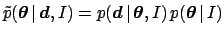. The difficult task is to normalize this function and to calculate all expectations in which we are interested, such as expected values, variances, covariances and other moments. We might also want to get marginal distributions, credibility intervals (or hypervolumes) and so on. As is well-known, if we were able to sample the posterior (even the un-normalized one), i.e. to generate points of the parameter space according to their probability, we would have solved the problem, at least approximately. For example, the one-dimensional histogram of parameter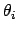would represent its marginal and would allow the calculation of,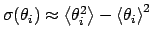and of probability intervals (in the previous formulae stand for arithmetic averages of the MC sample).

Let us consider the probability functionof the discrete variables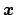and a function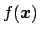of which we want to evaluate the expectation over the distribution. Extending the one-dimensional formula of Tab. 1 to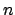dimension we have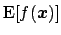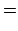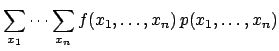(108)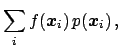(109)

where the summation in Eq. (109) is over the components, while the summation in Eq. (110) is over possible points in the-dimensional space of the variables. The result is the same.

If we are able to sample a large number of points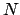according to the probability function, we expect each point to be generatedtimes. The average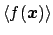, calculated from the sample as(110)

(in which the index is namedas a reminder that this is a sum over a time' sequence) can also be rewritten as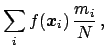(111)

just grouping together the outcomes giving the same. For a very large, the ratiosare expected to be very close' to(Bernoulli's theorem), and thusbecomes a good approximation of. In fact, this approximation can be good (within tolerable errors) even if not allare large and, indeed, even if many of them are null. Moreover, the same procedure can be extended to the continuum, in which case `all points' (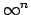) can never be sampled.

For simple distributions there are well-known standard techniques for generating pseudo-random numbers starting from pseudo-random numbers distributed uniformly between 0 and 1 (computer libraries are available for sampling points according to the most common distributions). We shall not enter into these basic techniques, but will concentrate instead on the calculation of expectations in more complicated cases.Next: Rejection sampling Up: Monte Carlo methods Previous: Monte Carlo methods
Giulio D'Agostini 2003-05-13